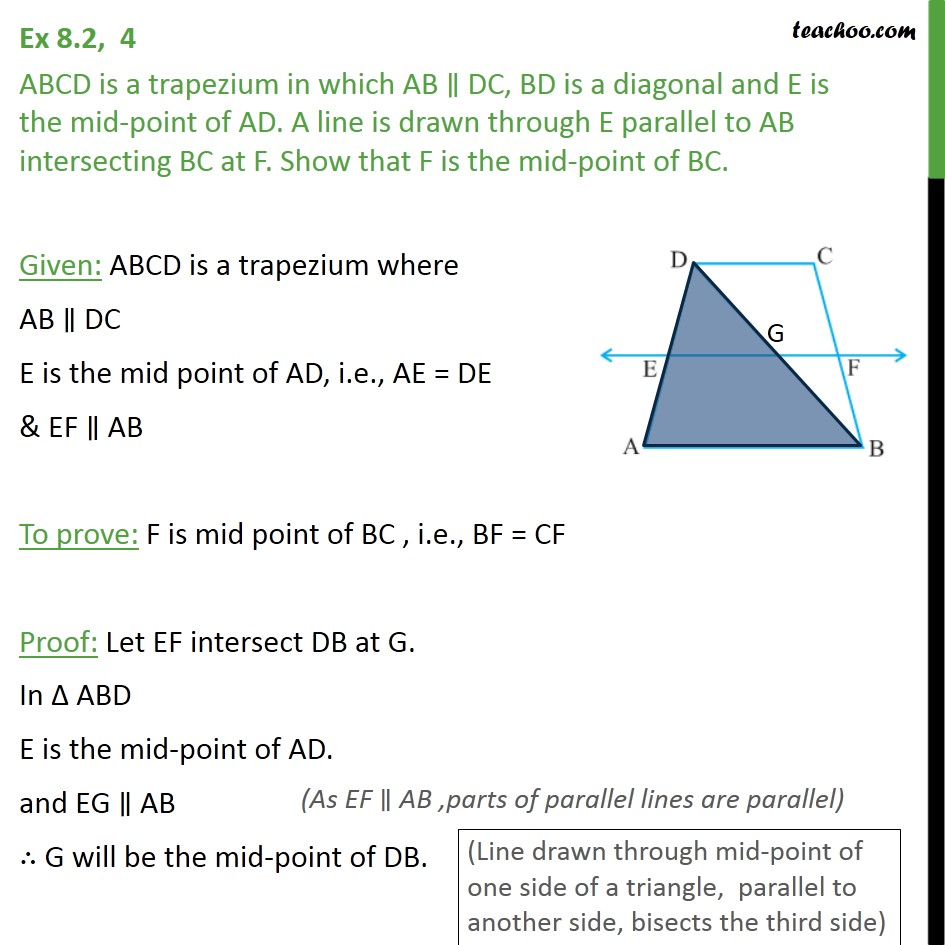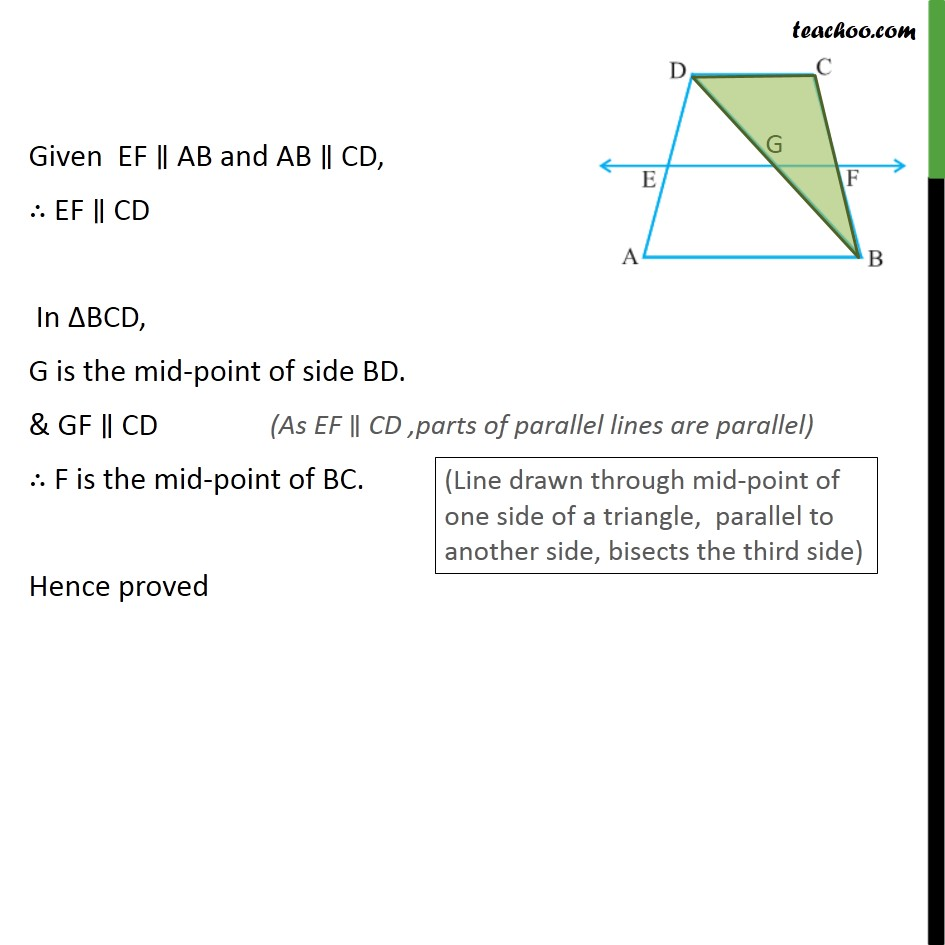Subscribe to our Youtube Channel - https://you.tube/teachoo

1. Chapter 8 Class 9 Quadrilaterals
2. Concept wise
3. Mid point theorem

Transcript

Ex 8.2, 4 ABCD is a trapezium in which AB ∥ DC, BD is a diagonal and E is the mid-point of AD. A line is drawn through E parallel to AB intersecting BC at F. Show that F is the mid-point of BC. Given: ABCD is a trapezium where AB ∥ DC E is the mid point of AD, i.e., AE = DE & EF ∥ AB To prove: F is mid point of BC , i.e., BF = CF Proof: Let EF intersect DB at G. In ∆ ABD E is the mid-point of AD. and EG ∥ AB ∴ G will be the mid-point of DB. Given EF ∥ AB and AB ∥ CD, ∴ EF ∥ CD In ΔBCD, G is the mid-point of side BD. & GF ∥ CD ∴ F is the mid-point of BC. Hence proved

Mid point theorem

About the AuthorDavneet Singh
Davneet Singh is a graduate from Indian Institute of Technology, Kanpur. He has been teaching from the past 9 years. He provides courses for Maths and Science at Teachoo.